Perpendicular Lines

Learning Objective(s)

·         Define perpendicular lines.

·         Recognize and create graphs and equations of perpendicular lines.

Introduction

Perpendicular lines are two or more lines that intersect at a 90-degree angle, like the two lines drawn on this graph, and the x and y axes that orient them.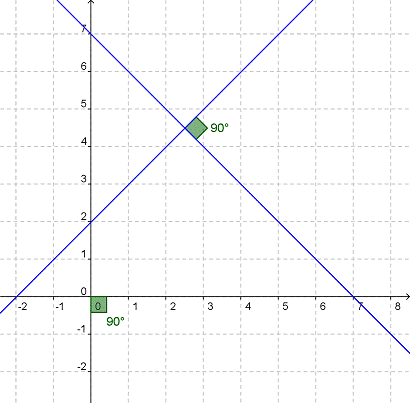Perpendicular lines are everywhere, not just on graph paper but also in the world around us, from the crossing pattern of roads at an intersection to the colored lines of a plaid shirt. In our daily lives, we may be happy to call two lines perpendicular if they merely seem to be at right angles to one another. In algebra, we use equations to make sure of it.

Recognizing Perpendicular Lines

Let’s compare the equations of lines that are perpendicular and those that are not to see if there is a pattern that will allow us to recognize perpendicular lines. We’ll start with two lines that we know are not perpendicular.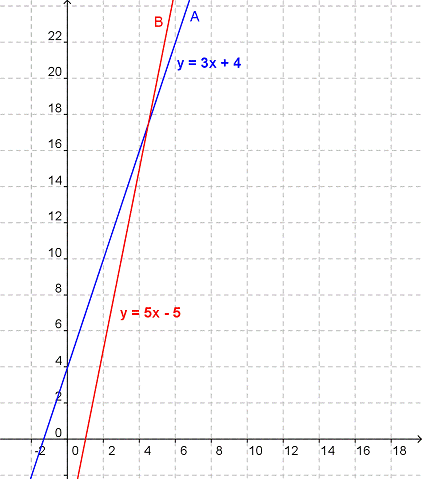Line A has an equation of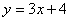, and Line B has an equation of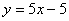.  These two lines are clearly not at 90-degrees to one another. Do you see any connection between their equations?

If you couldn’t find anything—you’re right. There isn’t any link between the slopes or [y-intercepts] of these lines.

Now let’s look at two lines that are perpendicular: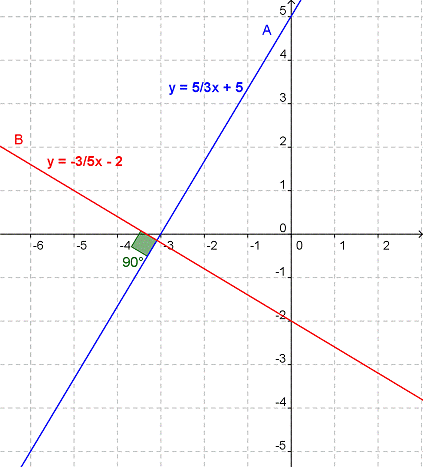On this graph, Line A has the equation ofand Line B has the equation of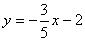.  Do you see any connection between these equations?

If you said no again this time, you missed something. It’s a little tricky, but there is a relationship between the slopes of these two lines. The slope of Line A is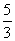, and the slope of Line B is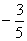. These numbers are opposite reciprocals. An opposite reciprocal is the fraction form of a number flipped upside down (that’s the reciprocal part), and with its sign changed (that’s the opposite part). Write those slopes side by side and it becomes more obvious:andare opposite reciprocals.

This is the defining feature of the equations of perpendicular lines—the slopes of perpendicular lines are opposite reciprocals.

If a line has a slope of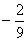, what is the slope of a line perpendicular to it?

A)B)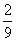C)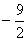D)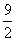Show/Hide Answer

Creating Perpendicular Lines

Now that we’ve learned what perpendicular lines have in common, let’s see if we can use that knowledge to create the perpendicular to a given line. Spend a few moments moving the red line on the graph below, and see if you can make it perpendicular to the blue line. The slider bar marked m will let you manipulate the slope. First, figure out the slope of the blue line, then change the value of m to the opposite reciprocal of that number. Do the lines look perpendicular to you now?

Now try changing the value of b, which is the y-intercept of the red line. Do you see that there are an infinite number of perpendicular lines? It doesn’t matter what the y-intercepts are, lines are perpendicular as long their slopes are opposite reciprocals of one another.

To create a perpendicular line from an equation, we once again start by identifying the slope of the original line. Then we write a second equation, changing the slope to the opposite reciprocal of the slope in the original equation.

Which of the following lines are perpendicular to the line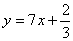?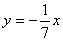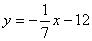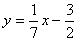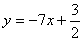A)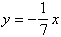andB)andC)D) all of the lines are perpendicular

Show/Hide Answer

Summary

Perpendicular lines are lines that cross one another at a 90° angle. They have slopes that are opposite reciprocals of one another. Unlike parallel lines that never touch, perpendicular lines must intersect.